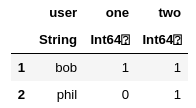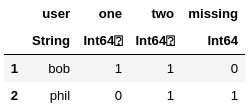# How do I not skip missing values when using stack / unstack for pivot tables

Hi how’s it going,

Here’s an example to better explain what I mean:

``````df = DataFrame(:user=>["bob","bob","phil","phil"],:count=>["one","two","two",missing])
var1 = :user
var2 = :count

long_df = by(df,[var1,var2],var2=>length)
wide_df = unstack(long_df,var2,Symbol(var2,:_length))

for col in names(wide_df)[2:end]
wide_df[ismissing.(wide_df[col]), col] = 0
end
``````

returnswhereas I need it to returnhow would I go about doing this?

Thank you.

1 Like

So.

Firstly, FreqTables.jl were designed to exactly handle the case you have:

``````julia> using FreqTables

julia> freqtable(df, var1, var2)
2×3 Named Array{Int64,2}
user ╲ count │     one      two  missing
─────────────┼──────────────────────────
bob          │       1        1        0
phil         │       0        1        1
``````

the benefit is that you can go for more than two dimensions.

Now, if you want to stick to DataFrames.jl just do:

``````julia> combine(groupby(df, var1), [var2 => (x -> count(isequal(u), x)) => string(u) for u in unique(df[!, var2])])
2×4 DataFrame
│ Row │ user   │ one   │ two   │ missing │
│     │ String │ Int64 │ Int64 │ Int64   │
├─────┼────────┼───────┼───────┼─────────┤
│ 1   │ bob    │ 1     │ 1     │ 0       │
│ 2   │ phil   │ 0     │ 1     │ 1       │
``````

Finally if you wanted to start with `long_df` you can do (but indeed it is cumbersome):

``````julia> coalesce.(unstack(transform(long_df, var2 => ByRow(string) => var2), var2, Symbol(var2, "_length")), 0)
2×4 DataFrame
│ Row │ user   │ missing │ one   │ two   │
│     │ String │ Int64   │ Int64 │ Int64 │
├─────┼────────┼─────────┼───────┼───────┤
│ 1   │ bob    │ 0       │ 1     │ 1     │
│ 2   │ phil   │ 1       │ 0     │ 1     │
``````

I think the easiest thing would just be to impute some value and rename the column after, which is cumbersome for sure. Maybe file an issue for a feature request so this doesn’t get lost.

No guarantee it will be implemented, but we can discuss the merits in an issue.